# The Sort Functions in Excel (SORT, and SORTBY)

in hive-102332 •  last month  (edited)

In this article we are going to explore the SORT functions in Excel.

Included in the new Dynamic Arrays functions are 2 Sort functions (SORT and SORTBY) and the UNIQUE function.  UNIQUE is often used in conjunction with the SORT functions. And both of the SORT functions have different use cases.

If you are not yet familiar with Dynamic Arrays, I would recommend you check out this article here - Excel Dynamic Arrays, Changing how Excel works

Let’s take a look at each function first with some examples before you try and solve a problem using a combination of these new Dynamic array functions.

### SORT

The syntax for SORT is

=SORT(array, [sort index], [sort order], [sort col])

Where

Array is the data you want to sort

Sort index is the column number you want to sort by

Sort order allows you select between ascending and descending.

And Sort Col allows you select between True to sort by column and False to sort by row.

The SORT is used when you want to sort data by one or more columns (or rows) and it returns a dynamic array of values

#### SORT Example 1 – Sort by 1 column

Using the following table of data we need to sort the data based on Products in ascending order.  We do not need to include the date column.To do this we can use the formula

=SORT(B2:E19,1,1)

As the default for the sort column is column 1 and the default for sort order is ascending, we can also right the formula as

=SORT(B2:E19)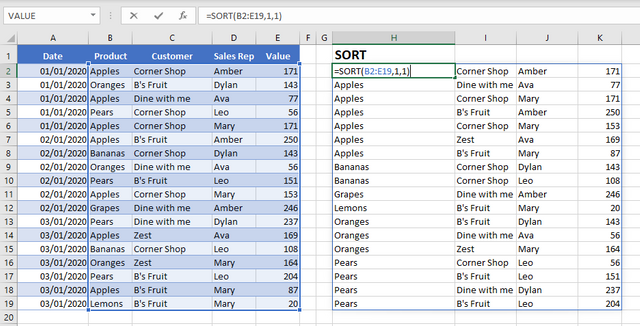#### SORT example 2: SORT by 2 or more columns

Using sort, we can also sort data by more than one column.  Lets sat we want to sort first by the Sales rep, then the Value and last by the customer, all in ascending order

To do this we would use the formula

=SORT(C2:E19,{3,2,1})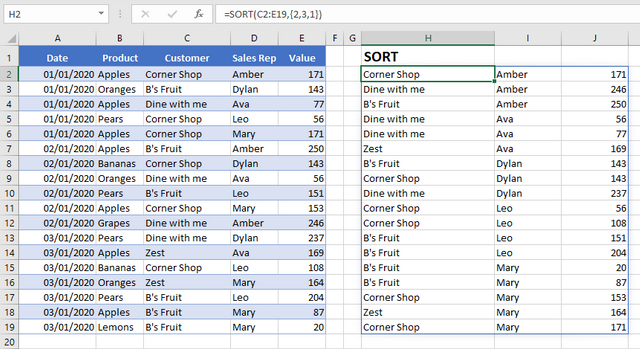It is important to note when we are sorting by multiple columns, the column numbers go inside {} in the order they have to be sorted.

Lets say you wanted a different sort order for each column, you would also include the sort orders within {} in the same order that you places the column numbers. For example, we want to sort the Values on descending order and the customer and sales rep in ascending order we would use the formula

=SORT(C2:E19,{2,3,1},{1,1,-1})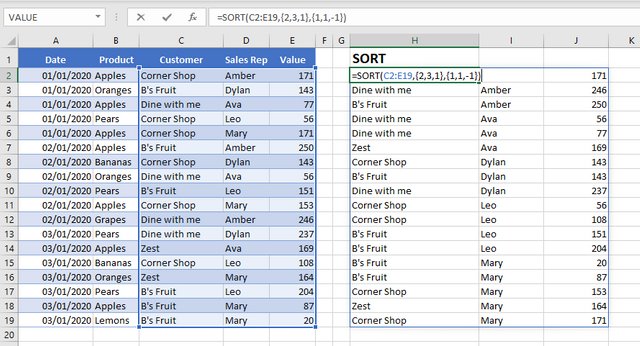The SORT function does exactly what it says on the tin.  It sorts an array of values.  However, when using the sort function the sort column must be contained within the sort array.  What if you wanted to sort an array based on a column that is not included?  In this case you would use SORTBY.

### SORTBY

The Syntax for sort by is

=SORYBY(array, by array 1, [sort order 1]…)

Where

Array are the values you want to sort

By array 1 is the first column or array you want to sort by

Sort order allows you select between ascending and descending

With the SORTBY function you can include multiple sort by arrays and you can state a different sort order for each sort array.

#### SORTBY Example

Lets say we want only the Product and Customer sorted by the products and then value. We would use the formula

=SORTBY(B2:C19,B2:B19,1,E2:E19,1)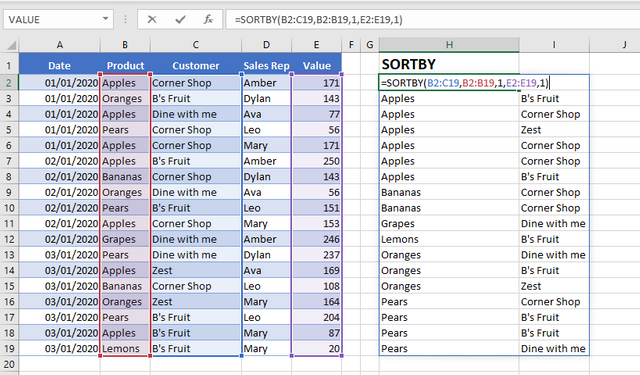In this case we have used the value column as the second sort array, although the value column is not part of the actual array being sorted.

### UNIQUE

The UNIQUE function returns a unique list, and it can return a list of unique items.

The syntax for UNIQUE is

=UNIQUE(array, [by column], [exactly once]

Where

Array is the data you want to find the unique values from

By column allows you to select between True for by column and False for by row

Exactly once allows you to select between True for values that occur only once and False for all distinct values.

To get a list of Unique products we would use the formula

=UNIQUE(B2:B19)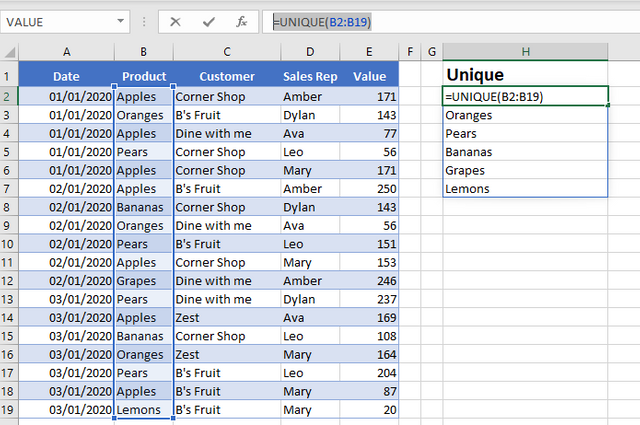However, to get a list of products, where the product only appears once we could use the formula

=UNIQUE(B2:B19,,TRUE)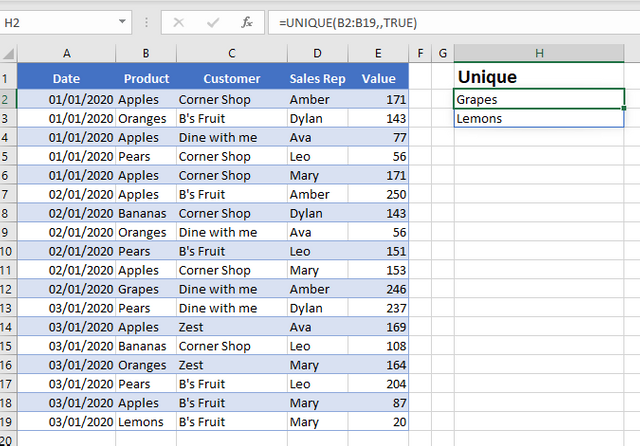### The Sort Functions in Excel - Your Turn

Now its your turn to try out the SORT functions in Excel.  The following table of data shows the running time for some members of a running club.  Using dynamic array functions, we wish to create a sorted list of unique team members based on the average running time, sorted from slowest to the fastest.  You can see the expected results in column D and E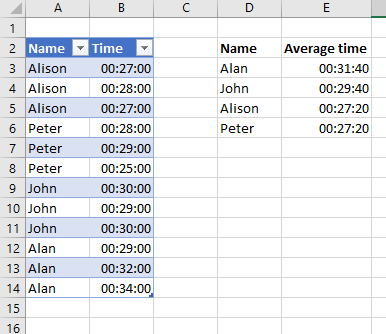What Dynamic array formulas would you use to re-create this?  Copy and paste the table of data below into Excel and try carry out this activity.  Pop your answers in the comments below.

 Name Time Alison 00:27:00 Alison 00:28:00 Alison 00:27:00 Peter 00:28:00 Peter 00:29:00 Peter 00:25:00 John 00:30:00 John 00:29:00 John 00:30:00 Alan 00:29:00 Alan 00:32:00 Alan 00:34:00
Hint:  The names and the time are two different formulas.  For the names try a combination of SORTBY, and UNIQUE and for the times try a combination of SORT and UNIQUE.  Both formulas also use the AVERAGEIFS function.

You will find the solution in the later half of this video.

### Take A FREE course with us Today!The Ultimate Excel Formulas Course

• includes XLOOKUP and will soon include Dynamic Arrays
Become a Power Pivot HeroGET and TRANSFORM DATA like a PRO

Power Query Excel 365

Learn DAX for Power Pivot and Power BI###### The Excel Club is the only Excel Blog in the world where you can Earn while you Learn Excel.  Find out about our Learn and Earn Activities now

Best Value Excel and Excel Power Tool Learning.  Access All Areas, Unlimited Learning SubscriptionSort Order:
·  18 days ago

Congratulations @theexcelclub! You have completed the following achievement on the Hive blockchain and have been rewarded with new badge(s) :You distributed more than 31000 upvotes. Your next target is to reach 32000 upvotes.

If you no longer want to receive notifications, reply to this comment with the word `STOP`Hive Whale - Make it spray and get your badge!